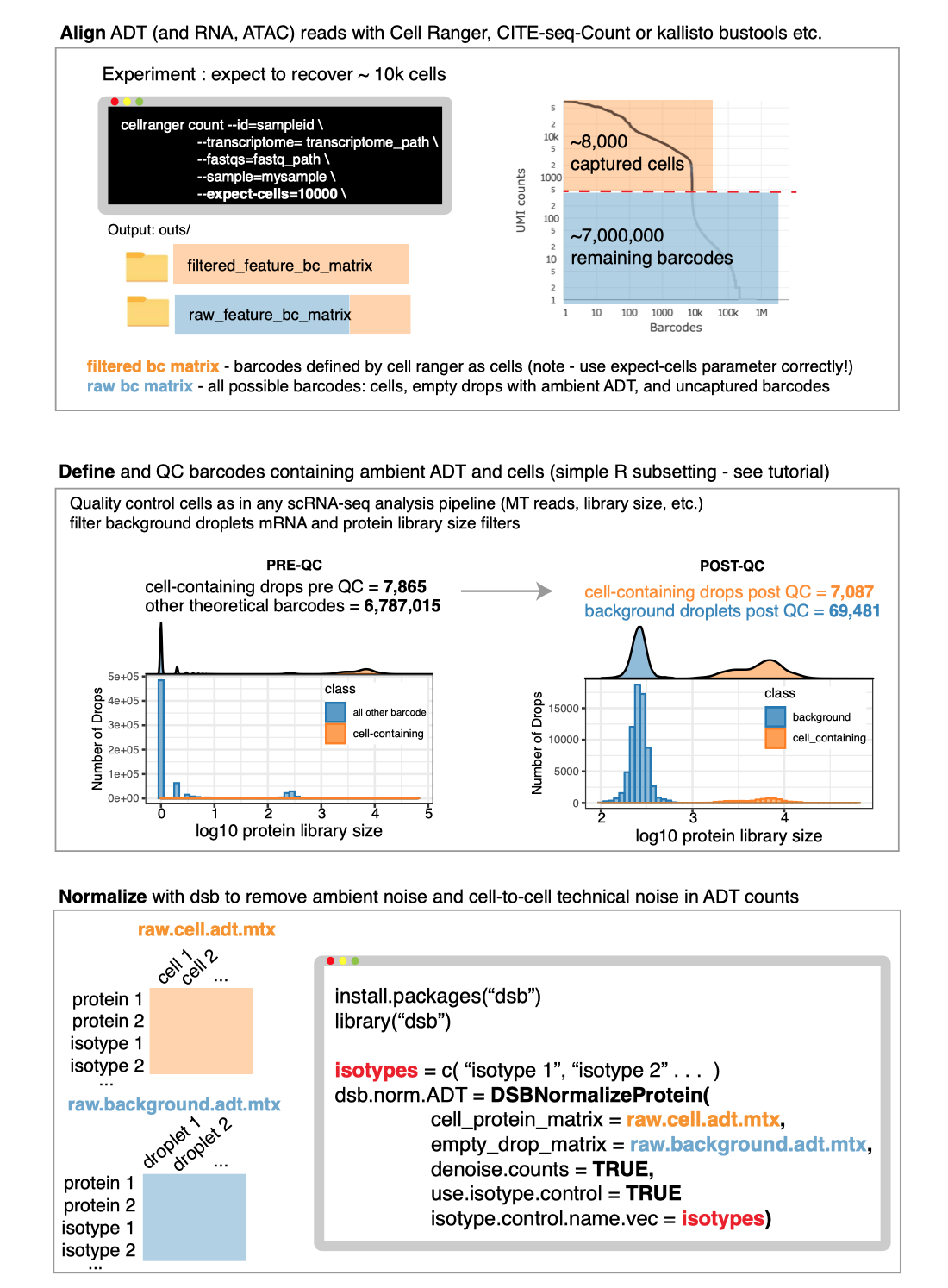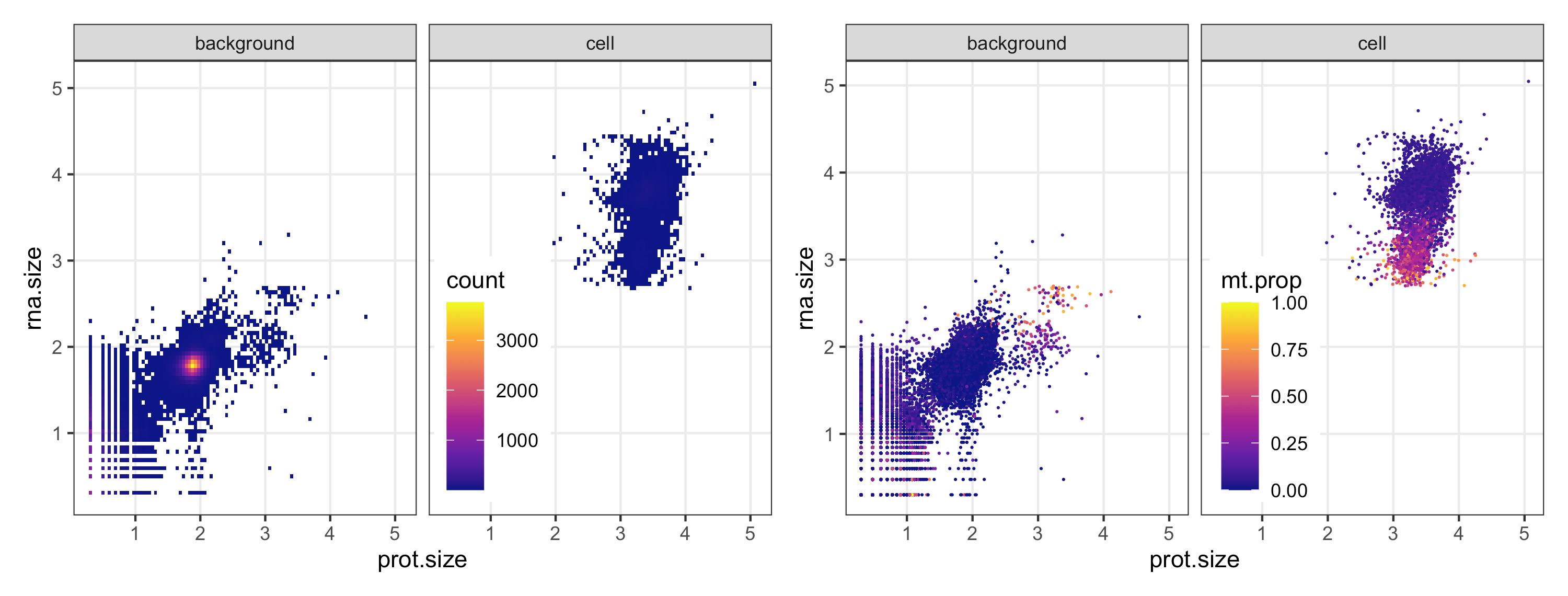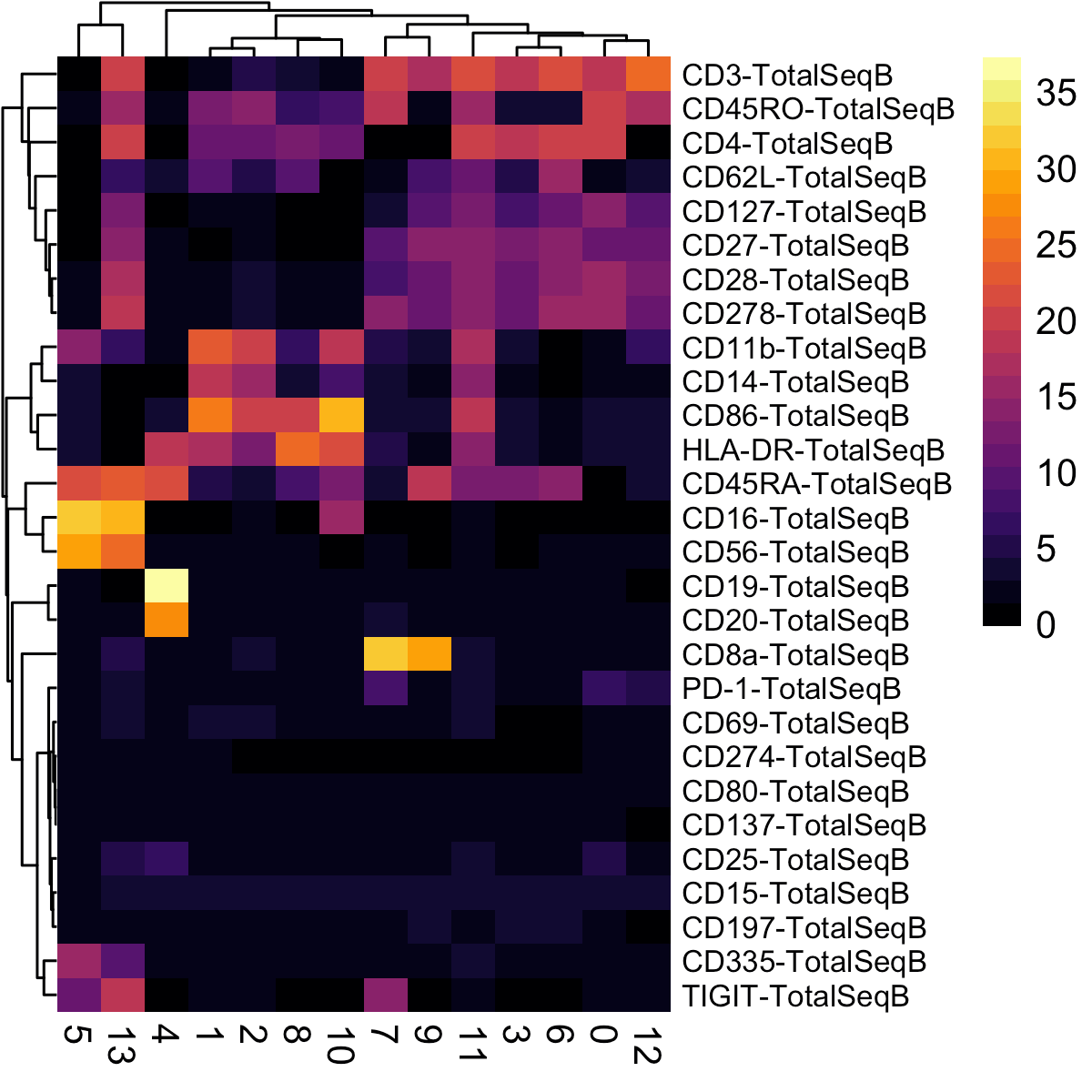Below we demonstrate an end-to-end basic CITE-seq analysis starting from UMI count alignment output files from Cell Ranger. Standard output files from Cell Ranger are perfectly set up to use dsb. Our method is also compatible with any alignment tool; see: using other alignment tools. We load unfiltered UMI data containing cells and empty droplets, perform QC on cells and background droplets, normalize with dsb, and demonstrate protein-based clustering and multimodal RNA+Protein joint clustering using dsb normalized values with Seurat’s Weighted Nearest Neighbor method.

Please cite the dsb manuscript if you used our software or found the experimental and modeling derivation of ADT noise sources in our paper helpful:
dsb manuscript

Recent publications using the dsb method
Recent Publications

Topics covered in other vignettes on CRAN: Integrating dsb with Bioconductor, integrating dsb with python/Scanpy, Using dsb with data lacking isotype controls, integrating dsb with sample multiplexing experiments, using dsb on data with multiple batches, advanced usage - using a different scale / standardization based on empty droplet levels, returning internal stats used by dsb, outlier clipping with the quantile.clipping argument, other FAQ.

## Background and motivation

Protein data derived from sequencing antibody derived tags (ADTs) in CITE-seq and other related assays has substantial background noise. Our paper outlines experiments and analysis designed to dissect sources of noise in ADT data we used to developed our method. We found all experiments measuring ADTs capture protein-specific background noise because ADT reads in empty / background drops (outnumbering cell-containing droplets > 10-fold in all experiments) were highly concordant with ADT levels in unstained spike-in cells. We therefore utilize background droplets which capture the ambient component of protein background noise to correct values in cells. We also remove technical cell-to-cell variations by defining each cell’s dsb “technical component”, a conservative adjustment factor derived by combining isotype control levels with each cell’s specific background level fitted with a single cell model.

## Installation and quick overview

The method is carried out in a single step with a call to the `DSBNormalizeProtein()` function.
`cells_citeseq_mtx` - a raw ADT count matrix `empty_drop_citeseq_mtx` - a raw ADT count matrix from non-cell containing empty / background droplets.
`denoise.counts = TRUE` - implement step II to define and remove the ‘technical component’ of each cell’s protein library.
`use.isotype.control = TRUE` - include isotype controls in the modeled dsb technical component.

``````install.packages('dsb')
library(dsb)

cell_protein_matrix = cells_citeseq_mtx,
empty_drop_matrix = empty_drop_citeseq_mtx,
denoise.counts = TRUE,
use.isotype.control = TRUE,
isotype.control.name.vec = rownames(cells_citeseq_mtx)[67:70]
)``````

Download BOTH the Feature / cell matrix (filtered) and Feature / cell matrix (raw) count matrices here: public 10X Genomics CITE-seq data.

## Step 1 A note on alignment of ADTs

Download BOTH the Feature / cell matrix (filtered) and Feature / cell matrix (raw) count matrices here: public 10X Genomics CITE-seq data.

To use dsb, we will load ADT and RNA data from cells and empty droplet aligned by Cell Ranger. dsb is also compatible with other aligners see: how to use Cite-Seq-Count and Kallisto with dsb.

## Step 1 A note on alignment of ADTs

The Cell Ranger `raw_feature_bc_matrix` includes every possible cell barcode (columns) x genes / ADT (rows); about 7 Million barcodes for the V3 assay. A very small subset of those columns in the `raw_feature_bc_matrix` contain cells–those are separately provided in the `filtered_feature_bc_matrix` file. Another subset of the `raw_feature_bc_matrix` contain empty droplets capturing ambient antibodies. Instead of discarding that valuable sequencing data, we extract empty droplets capturing ADT using the code below and use them with dsb. Also please see note on Cell Ranger filtered output.

update Cell Ranger `multi` can also be used instead of Cell Ranger `count` (whether or not you are sample multiplexing). The `nulti` alignment may improve cell vs background calling because it utilizes both ADT and RNA to define cells. If you use `multi`, the full output (background and estimated cells) is located in the following cell ranger output directory: `outs/multi/count/raw_feature_bc_matrix/`. The filtered output containing only the called cells is in: `outs/per_sample_outs/YOUR_ID_NAME/count/sample_feature_bc_matrix/`.

Here is a visual showing for the workflow we will follow starting from alignment and proceeding through QC and normalizationHere we use the convenience function from Seurat `Read10X` which will automatically detect multiple assays and create two element list `Gene Expression` and `Antibody Capture`.

``````library(dsb)

# define cell-containing barcodes and separate cells and empty drops
stained_cells = colnames(cells\$`Gene Expression`)
background = setdiff(colnames(raw\$`Gene Expression`), stained_cells)

# split the data into separate matrices for RNA and ADT
prot = raw\$`Antibody Capture`
rna = raw\$`Gene Expression```````

Now calculate some standard meta data for cells that we will use for quality control using standard approaches used in scRNAseq analysis pipelines.

``````# create metadata of droplet QC stats used in standard scRNAseq processing
mtgene = grep(pattern = "^MT-", rownames(rna), value = TRUE) # used below

md = data.frame(
rna.size = log10(Matrix::colSums(rna)),
prot.size = log10(Matrix::colSums(prot)),
n.gene = Matrix::colSums(rna > 0),
mt.prop = Matrix::colSums(rna[mtgene, ]) / Matrix::colSums(rna)
)
# add indicator for barcodes Cell Ranger called as cells
md\$drop.class = ifelse(rownames(md) %in% stained_cells, 'cell', 'background')

# remove barcodes with no evidence of capture in the experiment
md = md[md\$rna.size > 0 & md\$prot.size > 0, ]``````

we now have 103,075 barcodes in `md` with RNA and protein data only about 7,000 contain cells. With dsb we use a majority of this data instead of discarding everything except the cells.

## Step 3 Quality control cells and background droplets

``````ggplot(md, aes(x = log10(n.gene), y = prot.size )) +
theme_bw() +
geom_bin2d(bins = 300) +
scale_fill_viridis_c(option = "C") +
facet_wrap(~drop.class)``````

The right two plots show the density of droplets; yellow areas have more droplets = this clearly shows the major background droplet distribution in the first plot from the left (the yellow area of high density). In the third plot from the left, background drops are colored MT gene proportion so we can see some may be low-quality apoptotic cells. We next set upper and lower cutoffs on the background matrix library size. Note that as shown in Supplementary Fig 7, normalized values are robust to background thresholds used, so long as one does not omit the major background population.``````background_drops = rownames(
md[ md\$prot.size > 1.5 &
md\$prot.size < 3 &
md\$rna.size < 2.5, ]
)

More than 70,000 empty droplets are included after QC.

We next quality control cells in a similar manner with additional quality control metrics calculated as in any standard scRNAseq analysis, e.g. see Luecken et. al. 2019 Mol Syst Biol

``````
# calculate statistical thresholds for droplet filtering.
cellmd = md[md\$drop.class == 'cell', ]

# filter drops with + / - 3 median absolute deviations from the median library size
rna.lower = median(cellmd\$rna.size) - rna.mult
rna.upper = median(cellmd\$rna.size) + rna.mult
prot.lower = median(cellmd\$prot.size) - prot.mult
prot.upper = median(cellmd\$prot.size) + prot.mult

# filter rows based on droplet qualty control metrics
qc_cells = rownames(
cellmd[cellmd\$prot.size > prot.lower &
cellmd\$prot.size < prot.upper &
cellmd\$rna.size > rna.lower &
cellmd\$rna.size < rna.upper &
cellmd\$mt.prop < 0.14, ]
)``````

Check: are the number of cells passing QC in line with the expected recovery from the experiment?

``length(qc_cells)``

 4096

Yes. After quality control above we have 4096 cells which is in line with the ~5000 cells loaded in this experiment.

``````cell.adt.raw = as.matrix(prot[ , qc_cells])
cell.rna.raw = rna[ ,qc_cells]
cellmd = cellmd[qc_cells, ]``````

## Optional step; remove proteins without staining

Proteins without raw data in stained cells (below, the maximum UMI count for one protein was 4, an order of magnitude below even the isotype controls) can be removed from both matrices prior to normalization. In many cases, removing proteins is not necessary, but we recommend checking your raw data.

``````# flter

prot pmax
CD34_TotalSeqB 4
CD80_TotalSeqB 60
CD274_TotalSeqB 75
IgG2b_control_TotalSeqB 90

``````# remove the non staining protein

## Step 4 Normalize protein data with the DSBNormalizeProtein Function

We are now ready to use dsb to normalize and denoise the ADTs. We normalize the raw ADT matrix before creating a Seurat object since we also use the empty droplets.

The method is carried out in a single step with a call to the `DSBNormalizeProtein()` function.
`cells_citeseq_mtx` - the raw ADT UMI count matrix containing cells
`empty_drop_citeseq_mtx` - the raw ADT UMI count matrix containing background droplets
`denoise.counts` - we set to TRUE (the recommended default) to define and remove technical cell to cell variations. `isotype.control.name.vec` - a vector of the isotype control antibodies from the rownames of the raw ADT matrix defined from `rownames(cell.adt.raw)` (see vignettes for data without isotype controls). For data without isotype controls, see the vignette section Using dsb with data lacking isotype controls.

``````# define isotype controls
isotype.controls = c("IgG1_control_TotalSeqB", "IgG2a_control_TotalSeqB",
"IgG2b_control_TotalSeqB")

# normalize and denoise with dsb with
cells.dsb.norm = DSBNormalizeProtein(
denoise.counts = TRUE,
use.isotype.control = TRUE,
isotype.control.name.vec = isotype.controls
)
# note: normalization takes ~ 20 seconds
# system.time()
# user  system elapsed
#  20.799   0.209  21.783 ``````

The function returns a matrix of normalized protein values which can be integrated with any single cell analysis software. We provide an example with Seurat below.

Advanced users may want to examine internal stats used in dsb, in that case use `return.stats = TRUE`. If the range of values after normalization is large, this is often due to a few low or high magnitude outliers. The simplest way to address these outliers is to clip the maximum values by setting `quantile.clipping = TRUE`. Finally a different pseudocount can be used with `define.pseudocount = TRUE` and `pseudocount.use`. Please see the vignettes on CRAN to learn more about different parameters that can be used in dsb

``````# dsb with non-standard options
denoise.counts = TRUE,
use.isotype.control = TRUE,
define.pseudocount = TRUE,
pseudocount.use = 1,
scale.factor = 'mean_subtract',
quantile.clipping = TRUE,
quantile.clip = c(0.01, 0.99),
return.stats = TRUE
)``````

## Integrating dsb with Seurat

Create a Seurat object. Make sure to add the dsb normalized matrix `cell.adt.dsb` to the `data` slot, not the `counts` slot.

``````# Seurat workflow
library(Seurat)

# integrating with Seurat
stopifnot(isTRUE(all.equal(rownames(cellmd), colnames(cell.rna.raw))))

# create Seurat object note: min.cells is a gene filter, not a cell filter
s = Seurat::CreateSeuratObject(counts = cell.rna.raw,
meta.data = cellmd,
assay = "RNA",
min.cells = 20)

# add dsb normalized matrix "cell.adt.dsb" to the "CITE" data (not counts!) slot
s[["CITE"]] = Seurat::CreateAssayObject(data = cells.dsb.norm)``````

## Clustering cells based on dsb normalized protein using Seurat

Now we cluster cells based on dsb normalized protein levels. Similar to workflow used in our paper Kotliarov et al. 2020 we don’t cluster based on principal components from ADT, instead directly using the normalized values.

``````# define proteins to use in clustering (non-isptype controls)
prots = rownames(s@assays\$CITE@data)[1:28]

# cluster and run umap
s = Seurat::FindNeighbors(object = s, dims = NULL,assay = 'CITE',
features = prots, k.param = 30,
verbose = FALSE)

# direct graph clustering
s = Seurat::FindClusters(object = s, resolution = 1,
algorithm = 3,
graph.name = 'CITE_snn',
verbose = FALSE)
# umap (optional)
# s = Seurat::RunUMAP(object = s, assay = "CITE", features = prots,
#                     seed.use = 1990, min.dist = 0.2, n.neighbors = 30,
#                     verbose = FALSE)

# make results dataframe
d = cbind(s@meta.data,
as.data.frame(t(s@assays\$CITE@data))
# s@reductions\$umap@cell.embeddings)
)``````

To see if we recovered the expected cell populations, it is often more informative and interpretable to first look at the summarized (median or mean) protein expression in each cluster–we recommend doing this before trying to look at cells in 2-d visualization plots like umap.

## dsb derived cluster interpretation

dsb values are interpretable as the number of standard deviations of each protein from the expected noise (if using the default settings) with additional correction for cell to cell technical variations. One can use this to set a threshold across all proteins for positivity, e.g. expression below 3 or 4 can be deemed unexpressed. If normalized with the same parameters from dsb, the same threshold can be applied across multiple datasets.

``````library(magrittr)
# calculate the median protein expression separately for each cluster
dplyr::group_by(CITE_snn_res.1) %>%
dplyr::summarize_at(.vars = prots, .funs = median) %>%
tibble::remove_rownames() %>%
tibble::column_to_rownames("CITE_snn_res.1")
# plot a heatmap of the average dsb normalized values for each cluster
color = viridis::viridis(25, option = "B"),
fontsize_row = 8, border_color = NA)``````## Weighted Nearest Neighbor multimodal clustering using dsb normalized values with Seurat

The dsb normalized values can be used with the Weighted Nearest Neighbor multimodal clustering method. WNN is an excellent way to find fine-grained cell states, especially on datasets larger than this small example data selected for purposes of demonstration.

### Method 1 – Seurat WNN default with PCA on dsb normalized protein

``````# use pearson residuals as normalized values for pca
DefaultAssay(s) = "RNA"
s = NormalizeData(s, verbose = FALSE) %>%
FindVariableFeatures(selection.method = 'vst', verbose = FALSE) %>%
ScaleData(verbose = FALSE) %>%
RunPCA(verbose = FALSE)

# set up dsb values to use in WNN analysis (do not normalize with CLR, use dsb normalized values)
DefaultAssay(s) = "CITE"
VariableFeatures(s) = prots
s = s %>%
ScaleData() %>%
RunPCA(reduction.name = 'apca', verbose = FALSE)

# run WNN
s = FindMultiModalNeighbors(
s, reduction.list = list("pca", "apca"),
dims.list = list(1:30, 1:18),
modality.weight.name = "RNA.weight",
verbose = FALSE
)

# cluster
s <- FindClusters(s, graph.name = "wsnn",
algorithm = 3,
resolution = 1.5,
verbose = FALSE,
random.seed = 1990)``````

### Method 2– Seurat WNN with dsb normalized protein directly without PCA

Below we show a version of WNN where we directly use normalized protein values without PCA compression. We have found this procedure works well for smaller (e.g. less than 30) protein panels, whereas datasets with many cells generated with recently available pre-titrated panels consisting of more than 100 or 200 proteins may benefit more from dimensionality reduction with PCA.

``````## WNN with dsb values
# use RNA pearson residuals as normalized values for RNA pca
DefaultAssay(s) = "RNA"
s = NormalizeData(s, verbose = FALSE) %>%
FindVariableFeatures(selection.method = 'vst', verbose = FALSE) %>%
ScaleData(verbose = FALSE) %>%
RunPCA(verbose = FALSE)

# set up dsb values to use in WNN analysis
DefaultAssay(s) = "CITE"
VariableFeatures(s) = prots

# run true pca to initialize dr pca slot for WNN
## Not used {
s = ScaleData(s, assay = 'CITE', verbose = FALSE)
s = RunPCA(s, reduction.name = 'pdsb',features = VariableFeatures(s), verbose = FALSE)
# }

# make matrix of normalized protein values to add as dr embeddings
pseudo = t(GetAssayData(s,slot = 'data',assay = 'CITE'))[,1:29]
colnames(pseudo) = paste('pseudo', 1:29, sep = "_")
s@reductions\$pdsb@cell.embeddings = pseudo

# run WNN directly using dsb normalized values.
s = FindMultiModalNeighbors(
object = s,
reduction.list = list("pca", "pdsb"),
weighted.nn.name = "dsb_wnn",
knn.graph.name = "dsb_knn",
modality.weight.name = "dsb_weight",
snn.graph.name = "dsb_snn",
dims.list = list(1:30, 1:29),
verbose = FALSE
)

# cluster based on the join RNA and protein graph
s = FindClusters(s, graph.name = "dsb_knn",
algorithm = 3,
resolution = 1.5,
random.seed = 1990,
verbose = FALSE)``````

Defining marker genes for each cluster and visualizing aggregated per cluster expression

``````# create multimodal heatmap
vf = VariableFeatures(s,assay = "RNA")

# find marker genes for the joint clusters
Idents(s) = "dsb_knn_res.1.5"
DefaultAssay(s)  = "RNA"
rnade = FindAllMarkers(s, features = vf, only.pos = TRUE, verbose = FALSE)
dplyr::filter(avg_log2FC > 1 ) %>%
dplyr::group_by(cluster) %>%
dplyr::top_n(3) %\$% gene %>% unique

cite_data = GetAssayData(s,slot = 'data',assay = 'CITE') %>% t()
rna_subset = GetAssayData(s,assay = 'RNA',slot = 'data')[gene_plot, ] %>%
as.data.frame() %>%
t() %>%
as.matrix()

# combine into dataframe
d = cbind(s@meta.data, cite_data, rna_subset)

# calculate the median protein expression per cluster
dat_plot = d %>%
dplyr::group_by(dsb_knn_res.1.5) %>%
dplyr::summarize_at(.vars = c(prots, gene_plot), .funs = median) %>%
tibble::remove_rownames() %>%
tibble::column_to_rownames("dsb_knn_res.1.5") ``````

Next we can make a joint heatmap of protein and mRNA expression. It is helpful to visualize protein levels on the normalized dsb scale which was also used to cluster cells with relative mRNA expression as a z score.

``````# make a combined plot
suppressMessages(library(ComplexHeatmap)); ht_opt\$message = FALSE

# protein heatmap
# protein heatmap
prot_col = circlize::colorRamp2(breaks = seq(-1,25, by = 1),
colors = viridis::viridis(n = 27, option = "B"))
p1 = Heatmap(t(dat_plot)[prots, ],
name = "protein",
col = prot_col,
use_raster = T,
row_names_gp = gpar(color = "black", fontsize = 5)
)

# mRNA heatmap
mrna = t(dat_plot)[gene_plot, ]
rna_col = circlize::colorRamp2(breaks = c(-2,-1,0,1,2),
colors = colorspace::diverge_hsv(n = 5))
p2 = Heatmap(t(scale(t(mrna))),
name = "mRNA",
col = rna_col,
use_raster = T,
clustering_method_columns = 'average',
column_names_gp = gpar(color = "black", fontsize = 7),
row_names_gp = gpar(color = "black", fontsize = 5))

# combine heatmaps
ht_list = p1 %v% p2
draw(ht_list)``````